Attribute Gage Uncertainty – Part 2
Dr Jody Muelaner posted on September 10, 2019 |
Refining the resolution of calibrated samples, testing and fitting non-Gaussian probability.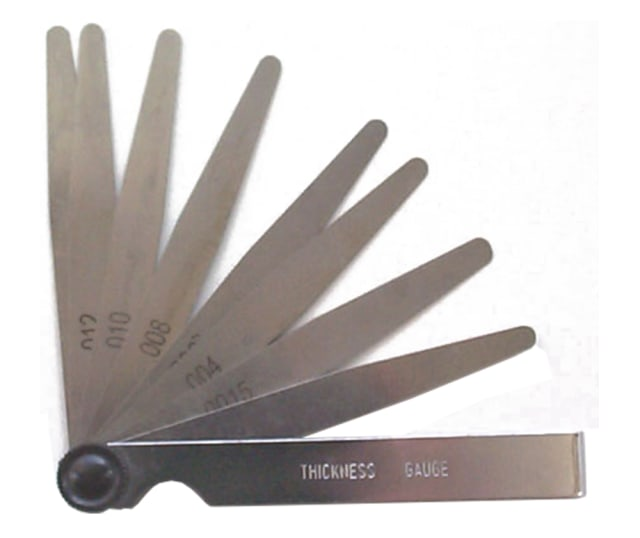Attribute gages are a type of measurement instrument or process which gives a binary pass/fail measurement result. Examples of attribute gages include go/no-go plug gages, feeler gages and many other types of special purpose hard gages. Many visual inspection processes may also be considered as attribute gages.Attribute gages are very commonly used in manufacturing for product verification. Understand the accuracy and capability of these measurements is therefore vital for a comprehensive understanding of quality in manufactured goods.

Uncertainty evaluation must consider all the quantities, or factors, that might influence the measurement result. The uncertainties of these individual influence quantities are first evaluated and then combined to give the uncertainty of the measurement result. Calculating the combined uncertainty requires a mathematical model that gives the sensitivity of the measurement result to changes in the influence quantities. This can be done using either an uncertainty budget or a numerical simulation. In most cases important influences to consider will include calibration uncertainty, repeatability, and environmental factors.

Determining the uncertainty for individual influence quantities can be carried out by making repeated measurements and performing a statistical evaluation of the results, usually to calculate the standard deviation. This is known as a Type-A evaluation. Repeatability is almost exclusively determined in this way. For other influence quantities, such as any calibration uncertainty, it may not be practical to evaluate the uncertainty in this way. Therefore some other method must be used, referred to as a Type-B evaluation. This could mean referencing an uncertainty value on a calibration certificate or even estimating the value in some cases.

In general, the evaluation of uncertainty of an attribute gage is the same as for a variable gage. The individual sources of uncertainty are evaluated and then combined using a mathematical model of the measurement. The key difference is in the way that repeatability is evaluated. Since attribute gages do not give a numerical result, it is not possible to directly calculate a standard deviation from a series of results. Instead, attribute gages simply give a pass or fail result. I explained the basics of evaluating uncertainty for an attribute gage in a previous article. It is possible to carry out a Type-A evaluation of the repeatability uncertainty for an attribute gage by first obtaining a number of calibrated reference parts that can be measured by the attribute gage. These references must have a range of values, close to the transitional value which separates pass from fail for the attribute gage. In theory, if a reference were exactly at this transitional value, an attribute gage could be expected to pass the reference 50% of the time and fail it 50% of the time. References that are significantly larger or smaller than the transitional value would be either passed or failed by 100% of measurements. References close to the transitional value will have some other frequency. For each reference, the conventional true value can be obtained by calibration, and the frequency at which the attribute gage passes it can be determined by repeated measurement. A cumulative probability density function can, therefore, be fitted to the test results to determine the actual transitional value for the gage and its repeatability expressed as a standard deviation.

The previous article presented the basic method but did not show how to refine the resolution of reference samples, determine the significance of bias, or fit non-Gaussian probability distributions.

### Example Attribute Gage

The examples in this article will be based on the same gage as the previous example – a ‘go’ plug gage used to test a 12 mm hole with an H8 tolerance (a diameter of between 12.000 mm and 12.027 mm). The ‘go’ end of the go/no-go gage should fit into a hole which is greater than 12 mm in diameter and should not fit into a hole smaller than 12 mm in diameter.

12 mm is the threshold value, so calibrated reference holes must be used which are close to this value. In the previous example, the range of references initially selected allowed a good fit to the probability distribution for the gage, and the analysis was relatively straightforward. Each reference was measured 25 times: the frequency with which the attribute gage passed it was recorded, and a plot of calibrated size against frequency was made. The Excel Solver was then used to fit a normal distribution to this frequency plot using least squares minimization. For further details, see the previous article.Fig. 1: Results of repeatability study given as pass rate (frequency) and normal distribution fitted by least squares minimization.

### Refining the Resolution of Calibrated References

Imagine what would have happened if a different set of calibrated references had been used. Suppose that the references were at 0.005 mm increments. The results would be likely to look something like this: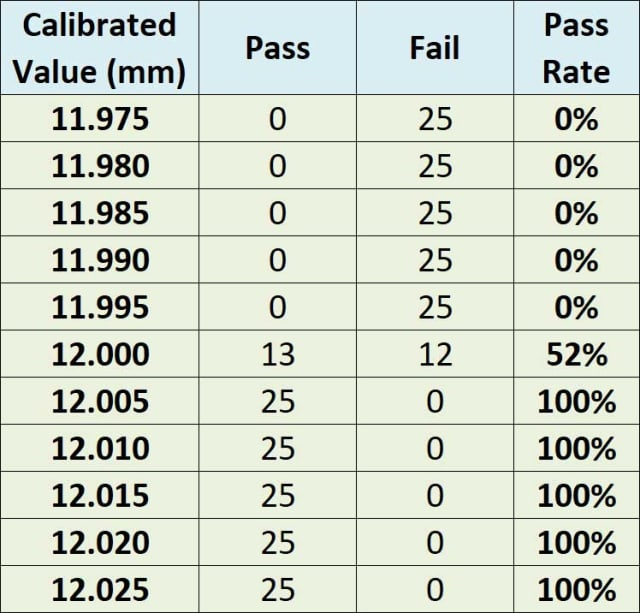Fig. 2: Results with calibrated references not spaced closely enough around the transitional value.

Clearly it would not be possible to obtain any meaningful estimate of the standard deviation from these results. It is clear that the standard deviation must be less than 0.005 but not much else can be determined, the standard deviation could just as easily be 0.002 or 0.00002. Carrying out a full gage study with this many samples was a complete waste of time because sensible increments had not yet been established.

The trick here is to subdivide the region between 0% pass and 100% pass until some more meaningful results are seen. There are various ways to approach this but probably the most efficient is with a binary search where the intervals are halved until something sensible is seen. Allowing for our resolution, we decide to try intervals of 0.003 next. There is no need to try so many values at this stage, since we are only trying to identify the approximate range of the distribution. This might produce the following results:Fig. 3: Results of binary search to find approximate limits of distribution.

It seems that we are getting an idea of the size of the distribution now but we might try a few more values to be sure:Fig. 4: Results with further references.

Based on these results we could safely proceed with a full attribute gage study, choosing reference values with 0.001 mm increments between 11.995 mm and 12.005 mm. This might produce the results seen in the previous example, to which a distribution can be fitted with confidence:Fig. 5: Attribute gage study results with well-chosen reference values allowing a distribution to be fitted with confidence.

It is also possible that we might initially start with values that are too close together to give any meaningful results. In this case you should expand the values. In many cases you will already have a good idea of the uncertainty of the gage you are studying and this can guide the initial selection of references. In any case it’s always best to test a few samples and experiment a bit in this way before investing the time in a full study.

### Determining the significance of bias in the gage

Before we can determine the significance of any bias in the gage we must first fit a distribution to the study results. This is carried out using regression, for example a least squares minimization as explained in Attribute Gage Uncertainty part one. A normal distribution has two parameters, mean and standard deviation, which are estimated in this process. The difference between the nominal dimension being measured by the gage (or the gage’s transitional value) and the mean for this fitted distribution is the bias. However, it is possible that this bias is not caused by any inherent bias in the gage but is simply a result of random variation in the gage. If the gage study were repeated, random variation would produce a slightly different set of results. The mean for the fitted distribution would, therefore, be slightly different. In the case where there is no inherent bias in the gage at all, these random variations would still produce some small bias

Based on the standard deviation and the number of measurements used to evaluate it, we can estimate how much random variation in the mean we would expect. This is called the standard error of the mean. If the bias we see in the results could be expected based purely on the standard error, then the bias is said to be not significant. If the bias is larger than this it is said to be significant.

Significant bias should be corrected, where possible. If the bias is not significant then you should not attempt to correct for it since you will be simply chasing random variations and may actually make the gage less accurate.

When dealing with values estimated by regression, determining standard errors can get quite complex since you may need to consider the standard error of the regression as well as the standard error of the mean. However, if we assume that we have obtained a good fit to our data then we don’t need to worry too much about that. The standard error of the mean is, given by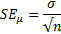In other words, it is the standard deviation divided by the square root of the number of repeat measurements used for each reference.

Typically if the bias is less than two standard errors of the mean it would not be considered significant (at 95% confidence).

### Fitting Different Probability Distributions

Often a normal or Gaussian distribution will explain the repeatability of a gage well but sometimes the random variation follows some other probability distribution. If you are unable to obtain a good fit to the normal distribution then it is worth first looking graphically at the distribution and then trying some other distribution that might provide a better fit.

When working with the normal distribution, Excel makes life easy by providing two different functions. We can work directly with the standard normal distribution, which has a mean of zero and a standard deviation of one, using the function:

=NORM.S.DIST(z,1)

This function has a single variable, z, which is the z-score or the number of standard deviations, and a flag which is set to 1 to give the cumulative probability density function. In the previous example we used a slightly different function for the parametric normal distribution with the mean and standard deviation as its two parameters. This meant that the value of the calibrated reference could be entered directly into the Excel function, together with the mean and the standard deviation.

To use the standard normal distribution, we could modify the previous example, so that the z-score is calculated from the calibrated reference (x), the mean, and the standard deviation. The z-score is given by: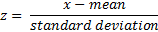Using this method,a column for the z-score can be added, and the standard normal distribution can then be used instead of the parametrized form. Taking this approach means that other distributions can also be used.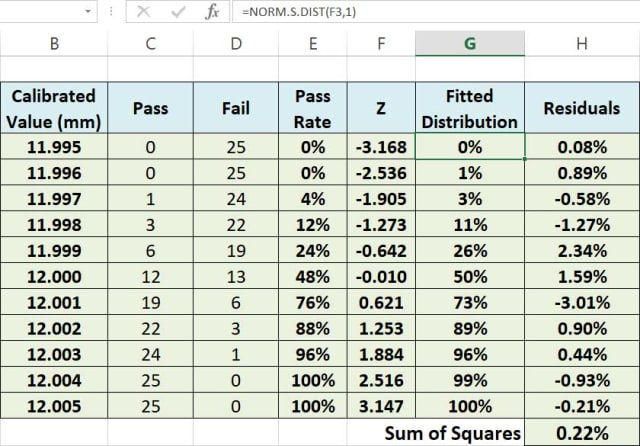Fig. 6: Column added for the z-score allowing the standard normal distribution to be used as well as other standard distributions.

The students t, or simply T distribution, is similar to the normal distribution but takes account of the increased uncertainty when small samples have been used to determine the standard deviation. With samples of less than 30, a T distribution should be used. This requires an additional parameter for the number of samples, n.

With the z-score now calculated, the fitted distribution column can use the formula for the T distribution in place of the one for the normal distribution.Fig. 7: Students T distribution fitted to the gage study data.

Some other distributions that may be relevant in gage studies include the exponential distribution, which provides the best estimate for non-negative quantities:

=EXPON.DIST(x,lambda,cumulative)

And the gamma distribution which is used where the measurement result is a number of objects counted:

=GAMMA.DIST(x,alpha,beta,cumulative) Gamma

This article has provided some more technical details on the important subject of attribute gage uncertainty. This builds on the previous article, which presented the basic method but did not show how to refine the resolution of reference samples, determine the significance of bias, or fit non-Gaussian probability distributions.

 Recommended For You